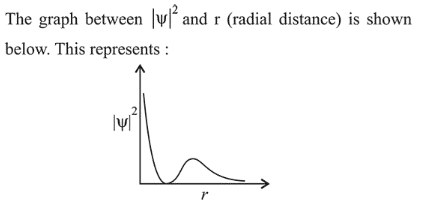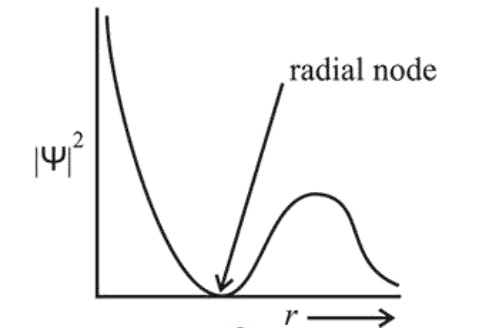# The graph between

Question:1. $3 s$ orbital

2. $2 s$ orbital

3. $1 s$ orbital

4. $2 p$ orbital

Correct Option: , 2

Solution:

The given probability density curve is for $2 s$ orbitaı due to the presence of only one radial node. $1 s$ and $2 p$ orbital do not have any radial node and $3 s$ orbital has two radial nodes. Hence, option (2) is correct.